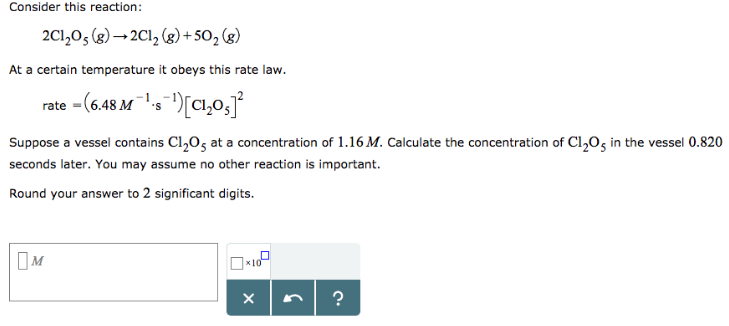# Problem: Consider this reaction: 2Cl2O5 (g) → 2Cl2 (g) + 5O2 (g) At a certain temperature it obeys this rate law. rate = (6.48 M-1 • s-1)[Cl2O5]2 Suppose a vessel contains Cl2O5 at a concentration of 1.16 M. Calculate the concentration of Cl2O5 in the vessel 0.820 seconds later. You may assume no other reaction is important. Round your answer to 2 significant digits.

###### FREE Expert Solution
97% (368 ratings)###### Problem Details

Consider this reaction:

2Cl2O5 (g) → 2Cl2 (g) + 5O2 (g)

At a certain temperature it obeys this rate law.

rate = (6.48 M-1 • s-1)[Cl2O5]2

Suppose a vessel contains Cl2O5 at a concentration of 1.16 M. Calculate the concentration of Cl2O5 in the vessel 0.820 seconds later. You may assume no other reaction is important.